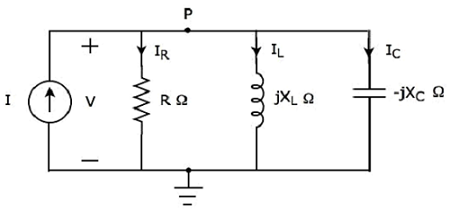# Network Theory - Parallel Resonance

In the previous chapter, we discussed the importance of series resonance. Now, let us discuss parallel resonance in RLC circuits.

## Parallel Resonance Circuit Diagram

If the resonance occurs in parallel RLC circuit, then it is called as Parallel Resonance. Consider the following parallel RLC circuit, which is represented in phasor domain.Here, the passive elements such as resistor, inductor and capacitor are connected in parallel. This entire combination is in parallel with the input sinusoidal current source.

Write nodal equation at node P.

$$- I + I_R + I_L + I_C = 0$$

$$\Rightarrow - I + \frac{V}{R} + \frac{V}{j X_L} + \frac{V}{-j X_C} = 0$$

$$\Rightarrow I = \frac{V}{R} - \frac{jV}{X_L} + \frac{jV}{X_C}$$

$\Rightarrow I = V[\frac{1}{R} + j \lgroup \frac{1}{X_C} - \frac{1}{X_L} \rgroup]$Equation 1

The above equation is in the form of I = VY.

Therefore, the admittance Y of parallel RLC circuit will be

$$Y = \frac{1}{R} + j \lgroup \frac{1}{X_C} - \frac{1}{X_L} \rgroup$$

## Parameters & Electrical Quantities at Resonance

Now, let us derive the values of parameters and electrical quantities at resonance of parallel RLC circuit one by one.

### Resonant Frequency

We know that the resonant frequency, fr is the frequency at which, resonance occurs. In parallel RLC circuit resonance occurs, when the imaginary term of admittance, Y is zero. i.e., the value of $\frac{1}{X_C} - \frac{1}{X_L}$ should be equal to zero

$$\Rightarrow \frac{1}{X_C} = \frac{1}{X_L}$$

$$\Rightarrow X_L = X_C$$

The above resonance condition is same as that of series RLC circuit. So, the resonant frequency, fr will be same in both series RLC circuit and parallel RLC circuit.

Therefore, the resonant frequency, fr of parallel RLC circuit is

$$f_r = \frac{1}{2 \pi \sqrt{LC}}$$

Where,

• L is the inductance of an inductor.
• C is the capacitance of a capacitor.

The resonant frequency, fr of parallel RLC circuit depends only on the inductance L and capacitance C. But, it is independent of resistance R.

We got the admittance Y of parallel RLC circuit as

$$Y = \frac{1}{R} + j \lgroup \frac{1}{X_C} - \frac{1}{X_L} \rgroup$$

Substitute, $X_L = X_C$ in the above equation.

$$Y = \frac{1}{R} + j \lgroup \frac{1}{X_C} - \frac{1}{X_C} \rgroup$$

$$\Rightarrow Y = \frac{1}{R} + j(0)$$

$$\Rightarrow Y = \frac{1}{R}$$

At resonance, the admittance, Y of parallel RLC circuit is equal to the reciprocal of the resistance, R. i.e., $\mathbf{\mathit{Y = \frac{1}{R}}}$

### Voltage across each Element

Substitute, $\frac{1}{X_C} - \frac{1}{X_L} = 0$ in Equation 1

$$I = V [\frac{1}{R} + j(0)]$$

$$\Rightarrow I = \frac{V}{R}$$

$$\Rightarrow V = IR$$

Therefore, the voltage across all the elements of parallel RLC circuit at resonance is V = IR.

At resonance, the admittance of parallel RLC circuit reaches to minimum value. Hence, maximum voltage is present across each element of this circuit at resonance.

### Current flowing through Resistor

The current flowing through resistor is

$$I_R = \frac{V}{R}$$

Substitute the value of V in the above equation.

$$I_R = \frac{IR}{R}$$

$$\Rightarrow I_R = I$$

Therefore, the current flowing through resistor at resonance is $\mathbf{\mathit{I_R = I}}$.

### Current flowing through Inductor

The current flowing through inductor is

$$I_L = \frac{V}{j X_L}$$

Substitute the value of V in the above equation.

$$I_L = \frac{IR}{j X_L}$$

$$\Rightarrow I_L = -j \lgroup \frac{R}{X_L} \rgroup I$$

$$\Rightarrow I_L = -jQI$$

Therefore, the current flowing through inductor at resonance is $I_L = -jQI$.

So, the magnitude of current flowing through inductor at resonance will be

$$|I_L| = QI$$

Where, Q is the Quality factor and its value is equal to $\frac{R}{X_L}$

### Current flowing through Capacitor

The current flowing through capacitor is

$$I_C = \frac{V}{-j X_C}$$

Substitute the value of V in the above equation.

$$I_C = \frac{IR}{-j X_C}$$

$$\Rightarrow I_C = j \lgroup \frac{R}{X_C} \rgroup I$$

$$\Rightarrow I_C = jQI$$

Therefore, the current flowing through capacitor at resonance is $I_C = jQI$

So, the magnitude of current flowing through capacitor at resonance will be

$$|I_C| = QI$$

Where, Q is the Quality factor and its value is equal to $\frac{R}{X_C}$

Note − Parallel resonance RLC circuit is called as current magnification circuit. Because, the magnitude of current flowing through inductor and capacitor is equal to Q times the input sinusoidal current I.